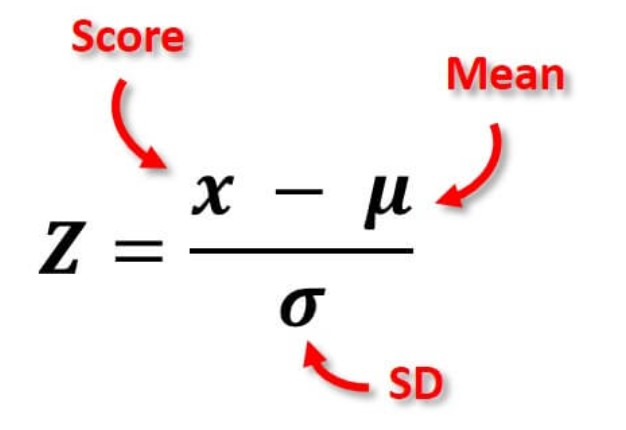# Z Score Calculator

Enter raw score, population mean, and standard deviation to find the z score using z score calculator.

Give Us Feedback

## Z Score Calculator

Z score calculator is an online tool that finds the z score of a normal distribution. It takes the population mean, standard deviation, and raw score as input to calculate z-score for a normal distribution.

Let’s find out the z-score definition, its formula, and how to calculate the z-score without using a z-score calculator.

## Definition - What is a z score?

Z score is the number of standard deviations by which the value of a raw score is above or below the mean value of what is being observed or measured. It is also known as the standard score.

Raw scores above the mean have positive standard scores, while those below the mean have negative standard scores. A z-score can be placed on a normal distribution curve. Z-scores range from -3 standard deviations up to +3 standard deviations.

## Z score formula

The following equation is used to calculate the z score.

Z = (x – μ) / σ## How to find the z score?

Z-score can also be calculated using the z value calculator above. In this section, we will find the z score using an example and its formula.

Example

Find the z-score if the standard deviation is 10, the population mean of the data is 20, and the raw score is 15.

Solution:

Step 1: Write down the given values.

x = 15

μ = 20

σ = 10

Step 2: Substitute the values in the z-score formula.

Z = (x – μ) / σ

Z = (15 – 20) / 10

Z = -5/10

Z = -0.5

### Math Tools